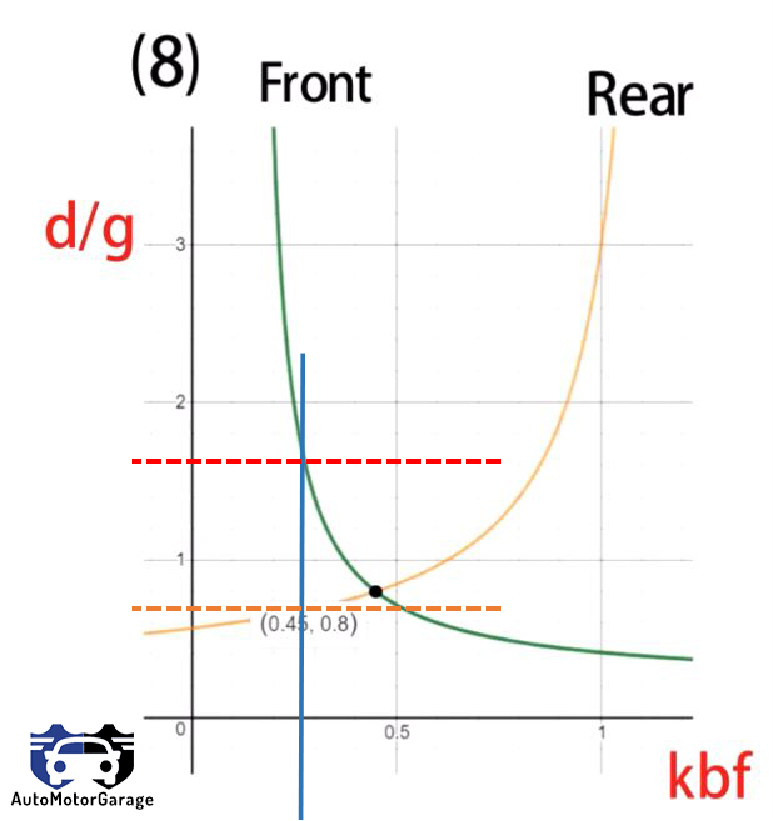top of page
Search

# What is the maximum value of deceleration and how it affects the Locking of Brakes ?

Drivers are asked to decelerate their vehicles from their maximum speed to zero speed in shortest time and their speed profiles are collected using Global Positioning System. Deceleration behavior of different vehicle types is significantly different. Vehicles with higher maximum speed have higher deceleration time, deceleration distance, maximum and mean deceleration rates during their deceleration manoeuvre. Deceleration also effects the locking of front and rear wheels. If the deceleration rate of front and rear tires is different,then they will lock differently.

Derivation for Maximum deceleration:-

Assumptions:-

• Aerodynamic force is neglected.For deriving the maximum deceleration for front and rear wheels , above figure will be considered.

Fbf - Front braking force by brakes

Fbr - Rear braking force by brakes

Rf - Front rolling resistance

Rr - Rear rolling resistance

h - height of COG

L - Wheelbase of car

L1 - distance of COG from front tire center

L2 - distance of COG form rear tire center

Balancing all forces parallel to ground,

Fbf+Fbr+Rf+Rr = (W/g)*d

Fb+fr*W=(W/g)*d (1)

Where,

Fb=Fbf +Fbr

R=Rf+Rr=fr*W(Total rolling resistance)

We know , Wf=L2*W/L + h/L[Fb+fr*W] (2) Wr=L1*W/L - h/L[Fb+fr*W] (3)

Putting equation (1) in (2) & (3) we get:-

Wf=[L2+(d/g)*h]*W/L (4) Wr=[L1-(d/g)*h]*W/L (5)

From equation (1),

Fb=(W/g)*d-fr*W

Fbf=kbf*Fb (Where kbf is the proportion in which braking force Fb is distributed in front wheels)

Fbf=kbf*W*[d/g-fr] (6)

Fbf(max)= μ*Wf (Where Fbf(max) is the maximum force generated between the front tire and ground contact)

For Locking of front wheels:-

Fbf(max)=Fbf

μ*Wf = kbf*W*[d/g-fr]

μ*[L2+(d/g)*h]*W/L=kbf*W*[d/g-fr]

(d/g)f=[μ*L2/L+kbf*fr]/[kbf-μ*h/L] (7) (Maximum deceleration in terms of g for front tire).

Similarly solving for rear tire:-

Fbr(max)=Fbr

μ*Wf=kbr*Fb

(d/g)r=[μ*L1/L+(1-kbf*fr)]/[(1-kbf)+μ*h/L] (8) (Maximum deceleration in terms of g for rear tire).

Fb =Fbf + Fbr (9)

Fbf=kbf*Fb (10) Fbr=kbr*Fb (11)

kbf+kbr=1 (12)

For understanding the effect of deceleration on locking of front and rear wheels , here i am assuming some values,

Let's assume:- h=1m L1= 3m L=4m L2= 1m μ=0.6 fr=0.2

Putting these values in equation (7) & (8) we get:-

(d/g)f =(0.15+0.2*kbf)/(kbf-0.15) (13)

(d/g)r =(0.65-0.2*kbf)/(1.15-kbf) (14)

Plotting the equation (13) & (14),On plotting equation (13) & (14) we get the following curve.This curve is between kbf & d/g.Green curve is for front tire and yellow curve is for rear tire. Intersection point of this curve is (0.45,0.8) , this is the point at which (d/g)f=(d/g)r. At this point both wheels will lock together with value of kbf=0.45 and value of kbr = 0.55(as kbf+kbr=1). The car will decelerate with deceleration of 0.8g .Now on increasing the value of kbf i.e I am increasing the value of Front braking force.

In the second figure of the curve you can see, the blue line is showing the increase value of kbf beyond 0.45 . The blue line is intersecting the front and rear curve both and is shown by red and orange line respectively. You can observe that the value of (d/g)r > (d/g)f and with the increase value of kbf we have more braking force at front and front wheels will lock first.Now on decreasing the value of kbf, which will increase the value of kbr (as kbf+kbr=1) i.e I am increasing the value of Rear braking force.In the third figure of the curve you can see, the blue line is showing the decrease value of kbf below 0.45 . The blue line is intersecting the front and rear curve both and is shown by red and orange line respectively. You can observe that the value of (d/g)f > (d/g)r and with the increase value of kbr we have more braking force at rear and rear wheels will lock first.

Note:- For understanding I assumed the value of μ=0.6 &fr=0.2 and we got the value of kbf=0.45 for ideal braking. The value of deceleration depends on the coefficient of friction μ ,So the value of (d/g) will change from road to road and also the locking of front and rear wheels will differ accordingly.

## Conclusion:-

• (d/g)f=[μ*L2/L+kbf*fr]/[kbf-μ*h/L] (Maximum deceleration in terms of g for front tire).

• (d/g)r=[μ*L1/L+(1-kbf*fr)]/[(1-kbf)+μ*h/L] (Maximum deceleration in terms of g for rear tire).

• When (d/g)f = (d/g)r , then both wheels will lock together.

• When (d/g)r > (d/g)f , then front wheels will lock first.

• When (d/g)f > (d/g)r , then rear wheels will lock first.